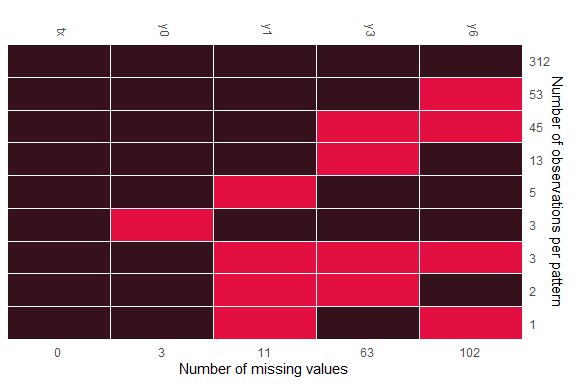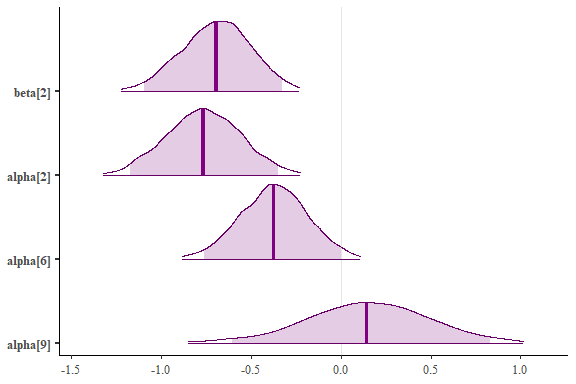## Example: Binary data imputation

In this example, we use the binary data from Schizophrenia data from NIMH study, i.e. `schizob`, which is included in the package. As described in Wang and Liu (2022), the original response variable was in numerical scale. The binary response was created using a cut-point of 3.5. The data is in a wide format. Please note that all binary variables which is set to be imputed should be converted into `factor` variables.

``````data(schizob)
head(schizob) %>% kbl(align = "c") %>%
kable_classic_2(full_width = F, html_font = "Cambria") %>%
column_spec(1, width = "2cm") %>%
add_header_above(c(" " = 1, "Responses at the baseline, week 1, week 3, and week 6" = 4))
``````
Responses at the baseline, week 1, week 3, and week 6
tx y0 y1 y3 y6
1 1 0 0 1
1 1 0 0 0
1 1 0 0 NA
1 0 0 0 0
0 1 1 1 1
1 1 1 1 1

Missing pattern is displayed in the following plot:Figure 1. Missing pattern of Schizophrenia data

To impute missing values with logit model, we can set up `family` argument, say, `family = binomial(link = "logit")`:

``````test = remiod(formula = y6 ~ tx + y0 + y1 + y3, data=schizob, family = binomial(link = "logit"),
trtvar = 'tx', algorithm = "jags", method = "MAR",
n.iter = 0, warn = FALSE, mess = FALSE)
print(test\$mc.mar\$models)

>                y6                   y3                   y1                   y0
"glm_binomial_logit" "glm_binomial_logit" "glm_binomial_logit" "glm_binomial_logit"
``````

However, if probit models are the choice, argument `models` must be set to accompany with `family` argument, like the following:

``````test.probit = remiod(formula = y6 ~ tx + y0 + y1 + y3, data=schizob, family = binomial(link = "probit"),
models = c(y0="glm_binomial_probit",y1="glm_binomial_probit",y3="glm_binomial_probit"),
trtvar = 'tx', algorithm = "jags", method = "MAR",
n.iter = 0, warn = FALSE, mess = FALSE)
print(test.probit\$mc.mar\$models)

>                    y6                    y3                    y1                     y0
"glm_binomial_probit" "glm_binomial_probit"  "glm_binomial_probit"  "glm_binomial_probit"
``````

Let’s run the Probit model with an adaptation of 10000 and 2000 iterations for 4 chains. Chains run in parallel, which is set through `doFuture` package:

``````registerDoFuture()
plan(multisession(workers = 4))

bp.test = remiod(formula=y6 ~ tx + y0 + y1 + y3, data=schizob, family = binomial(link="probit"),
models = c(y0="glm_binomial_probit",y1="glm_binomial_probit",y3="glm_binomial_probit"),
n.iter = 2000,  n.chains = 4, n.adapt = 10000, thin=1, mess=TRUE, warn=FALSE,
algorithm = "jags", trtvar = 'tx', method="MAR")

plan(sequential)
``````

The following plot show the estimated intervals as shaded areas under the posterior density curves for the parameters of treatment variable `tx` in imputation models:

• beta is the coefficient of tx in imputation model y6 ~ tx + y0 + y1 + y3;
• alpha is the coefficient of tx in imputation model y3 ~ tx + y0 + y1;
• alpha is the coefficient of tx in imputation model y1 ~ tx + y0;
• alpha is the coefficient of tx in imputation model y0 ~ tx.

The specified set of parameters can be submitted through argument `subset` with keyword `selected_parms` (alternatively, keyword `selected_vars`, which will be available in the new release, can also be used):

``````pms = c("beta","alpha","alpha","alpha")
mcsub = remiod:::get_subset(object = bp.test\$mc.mar, subset=c(selected_parms = list(pms)))

color_scheme_set("purple")
mcmc_areas(
mcsub,
pars = pms,
prob = 0.95, # 95% intervals
prob_outer = 0.99, # 99%
point_est = "mean"
)
``````Figure 2. Intervals under the estimated posterior density curves for coefficients of tx in imputation models

### Reference

Wang and Liu. 2022. “Remiod: Reference-Based Controlled Multiple Imputation of Longitudinal Binary and Ordinal Outcomes with Non-Ignorable Missingness.” arXiv 2203.02771.